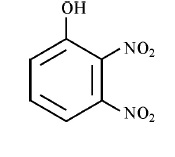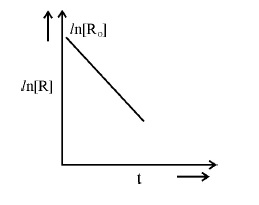NEW!  CBSE Papers PDF: Class-X, Class-XII

# CBSE Class-12 Question Papers for IOP/Comptt Examination 2017 : Delhi Scheme, Chemistry

Disclaimer: This website is not at associated with CBSE, For official website of CBSE visit - www.cbse.nic.in## CBSE Class-12 Question Papers for IOP/Comptt Examination 2017 : Chemistry, Set -1

CHEMISTRY (Theory)

Time allowed : 3 hours

Maximum Marks : 70

General Instructions :
(i) All questions are compulsory.
(ii) Questions number 1 to 5 are very short-answer questions and carry 1 mark each.
(iii) Questions number 6 to 10 are short answer questions and carry 2 marks each.
(iv) Questions number 11 to 22 are also short answer questions and carry 3 marks each.
(v) Question number 23 is a value based question and carry 4 marks.
(vi) Questions number 24 to 26 are long-answer questions and carry 5 marks each.
(vii) Use log tables, if necessary. Use of calculators is not allowed.

1. A metallic element crystallises into a lattice having a pattern of AB AB…… and packing of spheres leaves out voids in the lattice. What type of structure is formed by this arrangement ?

2. Which of the following is most effective in coagulating negatively charged hydrated ferric oxide sol ?
(i) NaNO3
(ii) MgSO4
(iii) AlCl3

3.Write IUPAC name of the following compound :5. What type of aldehydes undergo cannizaro reaction ?
6. Explain why on addition of 1 mol of glucose to 1 litre of water, the boiling point of water increases.

7.For a chemical reaction R-->P, variation in ln [R] vs time (t) plot is given below :For this reaction :
(i) Predict the order of reaction
(ii) What is the unit of rate constant (k) ?

8. “Orthophosphoric acid (H3PO4) is non-reducing whereas hypophosphorus acid (H3PO2) is a strong reducing agent.” Explain and justify the above statement with suitable example.
OR
(a) What is the covalence of nitrogen in N2O5 ?
(b) BiH3 is a stronger reducing agent than SbH3, why ?

9.Account for the following :
(i) The two oxygen-oxygen bond lengths in ozone molecule are identical.
(ii) Most of the reactions of fluorine are exothermic.